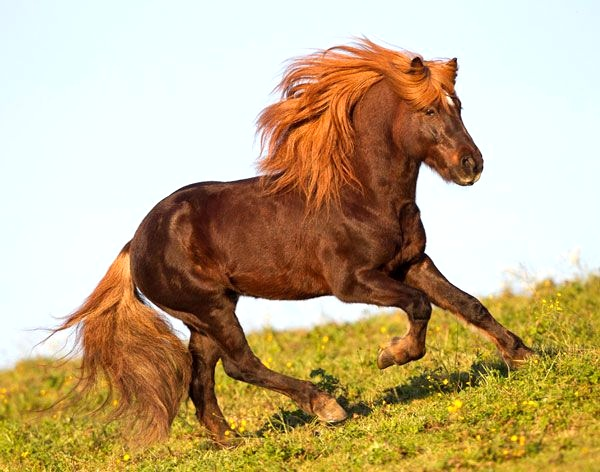Home » How are Horse Race Odds Payouts Calculated?

# How are Horse Race Odds Payouts Calculated?Horse racing is a thrilling and exciting sport that has the potential to bring in huge amounts of money if you make the right bets. However, the payouts can be confusing and intimidating, especially if you don’t know how they are calculated. In this article, we’ll discuss how horse race odds payouts are calculated and how understanding them can help you make better bets.

## What are Horse Racing Odds?

Horse racing odds are a representation of the probability of a horse winning a race. The higher the odds, the less likely it is for the horse to win. Odds can either be expressed in fractional or decimal form, with fractional odds being more popular in the UK and decimal odds being more popular in Europe and Australia.

Fractional odds are written as a fraction, with the numerator representing the profit you will make if you win the bet and the denominator representing the stake. For example, if you bet £5 at odds of 4/1, you would make a profit of £20 if you win (4 x £5 = £20).

Decimal odds represent the total amount of money that you will receive if you win the bet, including your stake. For example, if you bet £5 at odds of 5.0, you will receive a total of £25 (5 x £5 = £25).

## How are Horse Race Odds Payouts Calculated?

Horse race odds payouts are calculated by taking the odds of the horse and subtracting 1 from them. For example, if a horse has odds of 7.0, the payout would be calculated as 7.0 – 1 = 6.0. This means that if you bet £5 at these odds, you would receive a total of £30 (6 x £5 = £30).

## The Formula for Calculating Horse Race Odds Payouts

The formula for calculating horse race odds payouts is as follows:

Related content  How to Always Win at Horse Racing

## Odds Payout = (Odds – 1) x Stake

Where “Odds” is the odds of the horse and “Stake” is the amount of money you are betting.

For example, if you bet £5 at odds of 4.0, the payout would be calculated as (4.0 – 1) x £5 = £15.

## Factors Affecting Horse Race Odds Payouts

There are a number of factors that can affect the odds payouts in horse racing, such as the number of horses in the race, the track conditions, the form of the horses, and the jockeys. All of these factors can influence the odds and the payouts, so it’s important to take them into consideration before making a bet.

## The Type of Bet Placed

The type of bet placed can also affect the odds and the payouts. For example, a win bet pays out at the odds displayed, but a place bet pays out at a fraction of the odds and an each-way bet pays out at a fraction of the odds for both the win and the place.

## The Bookmaker’s Margin

The bookmaker’s margin is also an important factor to consider when calculating horse race odds payouts. The bookmaker’s margin is the amount of money the bookmaker takes from each bet, which is usually around 5% to 10%. This means that the odds and payouts displayed by the bookmaker are not the actual odds and payouts, as they have been reduced to account for the bookmaker’s margin.

## Conclusion

Horse race odds payouts can be complicated and intimidating, but understanding how they are calculated can help you make better bets. The formula for calculating horse race odds payouts is (Odds – 1) x Stake, but it’s important to take into consideration other factors such as the type of bet placed, the number of horses in the race, the track conditions, the form of the horses, the jockeys, and the bookmaker’s margin. By taking these factors into consideration, you can increase your chances of winning and make more profitable bets.